1595818440

# Neural Networks from Scratch - P.5 Hidden Layer Activation Functions

Neural Networks from Scratch book, access the draft now: https://nnfs.io

NNFSiX Github: https://github.com/Sentdex/NNfSiX

Spiral data function: https://gist.github.com/Sentdex/454cb

Python 3 basics: https://pythonprogramming.net/introdu
Intermediate Python (w/ OOP): https://pythonprogramming.net/introdu

#function

## Buddha Community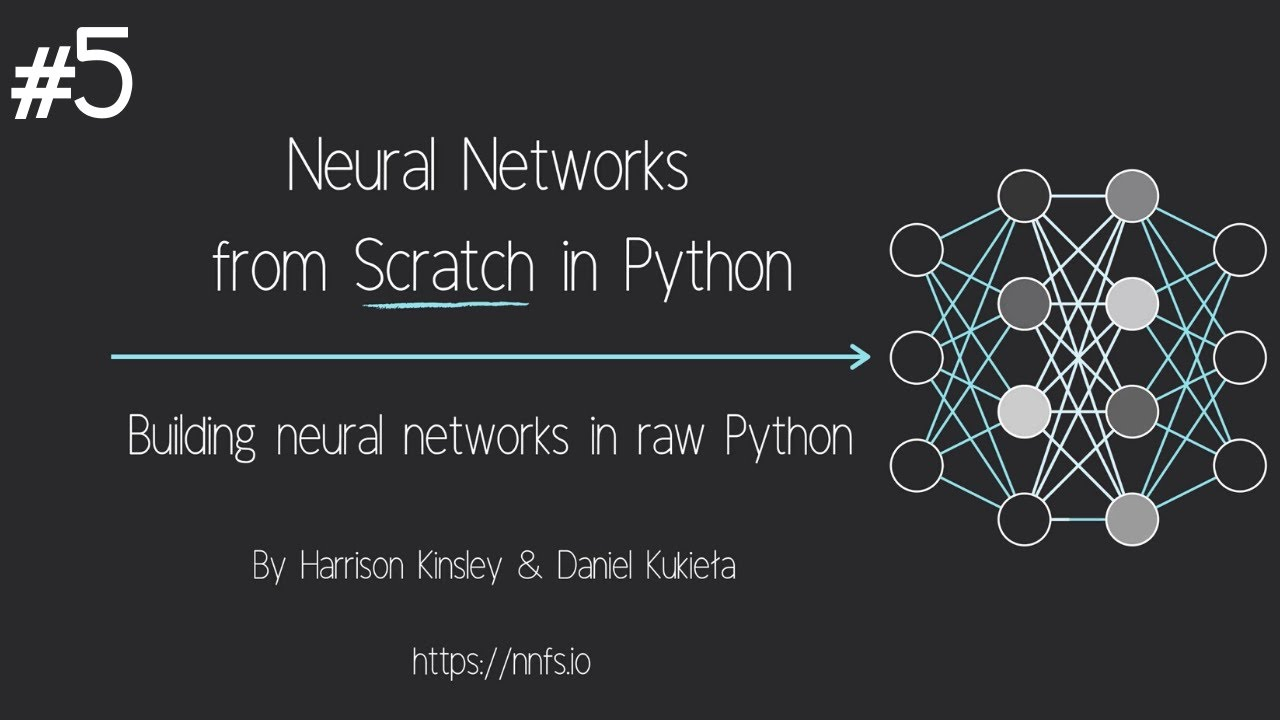1598034060

## Note

In this article I have discussed the various types of activation functions and what are the types of problems one might encounter while using each of them.

I would suggest to begin with a ReLU function and explore other functions as you move further. You can also design your own activation functions giving a non-linearity component to your network.

Recall that inputs x0,x1,x2,x3……xn and weights w0,w1,w2,w3………wn are multiplied and added with bias term to form our input.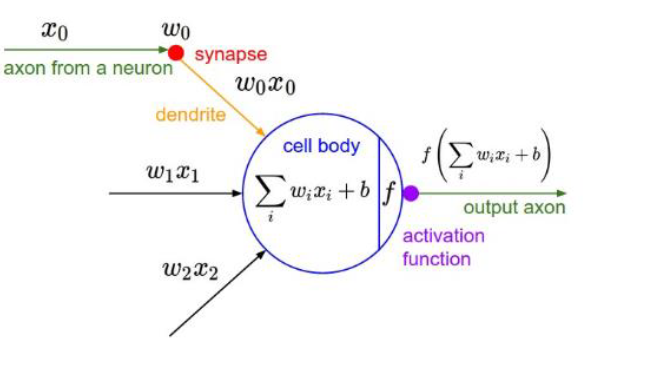Clearly W implies how much weight or strength we want to give our incoming input and we can think** b** as an offset value, making x*w have to reach an offset value before having an effect.

### As far we have seen the inputs so now what is activation function?

Activation function is used to set the boundaries for the overall output value.For Example:-let **z=X*w+b **be the output of the previous layer then it will be sent to the activation function for limit it’svalue between 0 and 1(if binary classification problem).

Finally, the output from the activation function moves to the next hidden layer and the same process is repeated. This forward movement of information is known as the forward propagation.

What if the output generated is far away from the actual value? Using the output from the forward propagation, error is calculated. Based on this error value, the weights and biases of the neurons are updated. This process is known as back-propagation.

#activation-functions #softmax #sigmoid-function #neural-networks #relu #function1596927420

## Activation Functions in Neural Network

Activation function, as name suggests, decides whether a neuron should be activated or not based on addition of a bias with the weighted sum of inputs. Hence, it is a very significant component of Deep Learning, as they in a way determine the output of models. Activation function has to be efficient so the model can scale along the increase in number of neurons.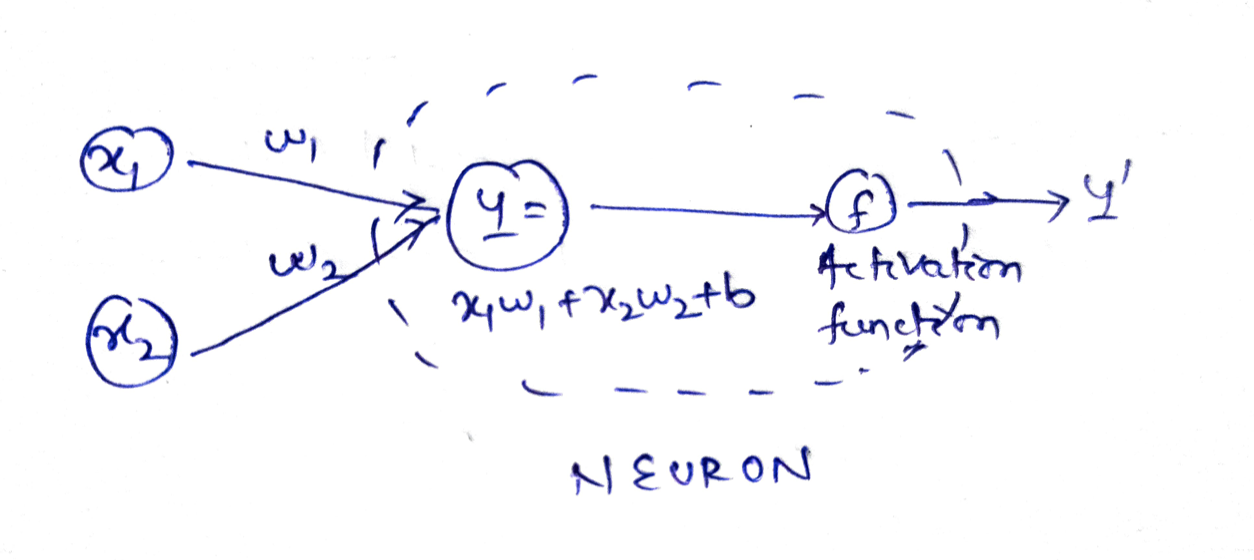To be precise, activation function decides how much information of the input relevant for the next stage.

For example, suppose x1 and x2 are two inputs with w1 and w2 their respective weights to the neuron. The output Y = activation_function(y).

Here, y = x1.w1 + x2.w2 + b i.e. weighted sum of inputs and bias.

Activation functions are mainly of 3 types. We will analyse the curves, pros and cons of each here. The input we work on will be a arithmetic progression in [-10, 10] with a constant difference of 0.1

``````x = tf.Variable(tf.range(-10, 10, 0.1), dtype=tf.float32)
``````

### Binary step

A binary step function is a threshold based activation function. If the input value is above or below a certain threshold, the neuron is activated and sends exactly the same signal to the next layer.

``````#Binary Step Activation
def binary_step(x):
return np.array([1 if each > 0 else 0 for each in list(x.numpy())])
do_plot(x.numpy(), binary_step(x), 'Binary Step')
``````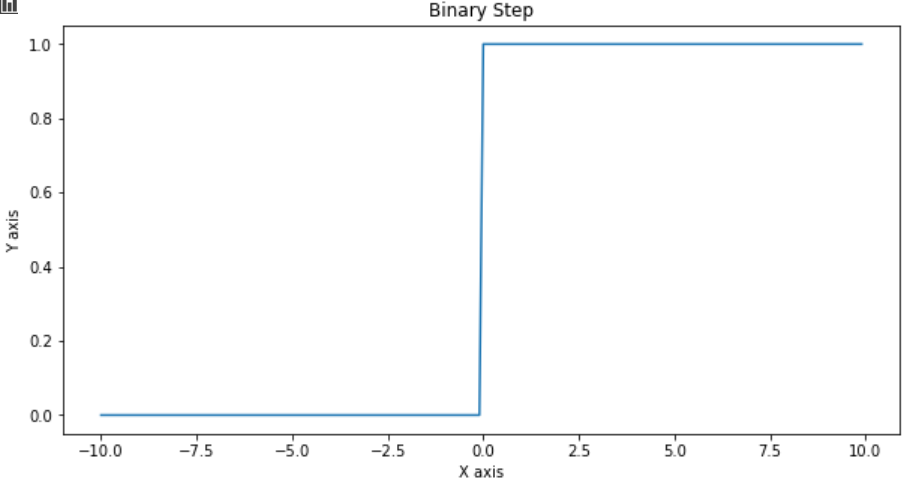Binary step is not used mostly because of two reasons. Firstly it allows only 2 outputs that doesn’t work for multi-class problems. Also, it doesn’t have a derivative.

### Linear

As the name suggests, the output is a linear function of the input i.e. y = cx

``````#Linear Activation
def linear_activation(x):
c = 0.1
return c*x.numpy()
do_plot(x.numpy(), linear_activation(x), 'Linear Activation')
``````

#activation-functions #artificial-intelligence #neural-networks #deep-learning #data-science #function1596300840

# Introduction

One of the biggest points of contention when designing a neural network is the configuration of the hidden nodes and layers in the model, i.e. how many hidden nodes and layers should be used for a given problem?

Many research papers have been written to address this issue, yet there remains no clear consensus as the answer to this question very much depends on the data being analysed.

The purpose of using hidden layers in the first place is to account for the variation in the output layers that is not fully captured by the features in the input layer.

However, determining the depth of the neural network (number of hidden layers) and the size of each layer (number of nodes) is somewhat an arbitrary process.

# Background

Let’s attempt to address this problem using an example: predicting average daily rates (ADR) for hotels. This is the output variable.

The features used in the model are as follows:

1. Cancellations: Whether a customer cancels their booking

2. Country of Origin

3. Market Segment

4. Deposit Paid

5. Customer Type

6. Required Car Parking Spaces

7. Arrival Date: Week Number

This analysis is based on the original study by Antonio, Almeida and Nunes (2016) as cited in the References section below.

# Neural Network Configurations

Given that there are **0 **values present for ADR (given that some customers cancel their hotel booking), as well as some negative values (possibly due to refunds), an ELU activation function is used.

Unlike RELU (which is a more standard activation function used in solving regression problems), ELU allows for negative outputs.

There is debate as to the number of hidden nodes that should be used in a hidden layer. For instance, this guide from Cross Validated illustrates several potential approaches to configuring the hidden nodes and layers.

One answer suggests calculating the number of nodes as being a value equal to or below the following in order to prevent overfitting: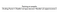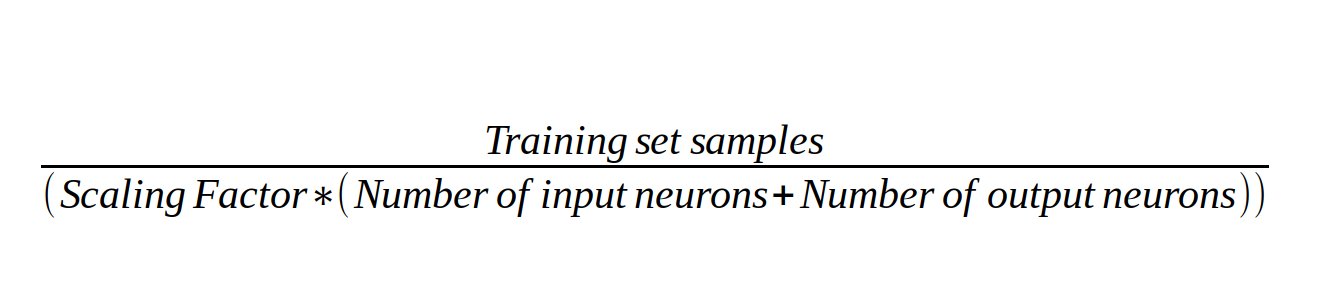Source: Image created by author — formula based on answer from Cross Validated

With 30,045 samples in our training set, a chosen factor of 2, as well as 7 input neurons and 1 output neuron — this gives 1,877 hidden nodes.

To test accuracy, three separate neural networks are run:

1. A network with 1 hidden layer and 1,877 hidden nodes
2. A network with 1 hidden layer and 4 hidden nodes
3. A network with 2 hidden layers and 4 hidden nodes each

# Neural Network 1 (1 hidden layer, 1,877 hidden nodes)

Using 1 hidden layer with 1,877 hidden nodes, such a neural network would be configured as follows:

``````Model: "sequential"
_________________________________________________________________
Layer (type)                 Output Shape              Param #
=================================================================
dense (Dense)                (None, 8)                 72
_________________________________________________________________
dense_1 (Dense)              (None, 1669)              15021
_________________________________________________________________
dense_2 (Dense)              (None, 1)                 1670
=================================================================
Total params: 16,763
Trainable params: 16,763
Non-trainable params: 0
_________________________________________________________________
``````

#hidden-layers #data-science #neural-networks #machine-learning #function1599233280

## Activation functions in Neural Networks

### What are Activation Functions?

Activation functions in neural networks are used to define the output of the neuron given the set of inputs. These are applied to the weighted sum of the inputs and transform them into output depending on the type of activation used.

Output of neuron = Activation(weighted sum of inputs + bias)

### Why we need Activation Functions?

The main idea behind using activation functions is to add non-linearity.

Now, the question arises why we need non-linearity? We need neural network models to **learn and represent complex functions. **Thus, using activation functions in neural networks, aids in process of learning complex patterns from data and adds the capability to generate non-linear mappings from inputs to outputs.

### Types of Activation Functions

1. Sigmoid- It limits the input value between 0 and 1.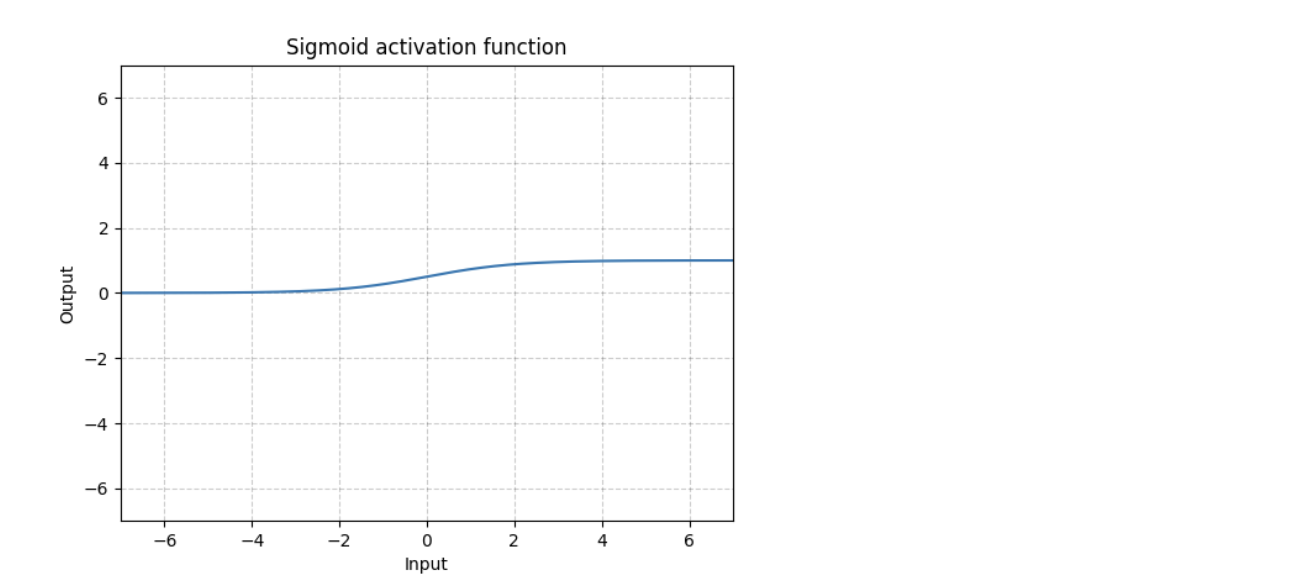Sigmoid maps the input to a small range of [0, 1]. As a result, there are large regions of the input space which are mapped to a very small range. This leads to a problem called vanishing gradient. It means that the most upstream layers will learn very slowly because essentially the computed gradient is very small due to the way the gradients are chained together.

2. Tanh- It limits the value between -1 and 1.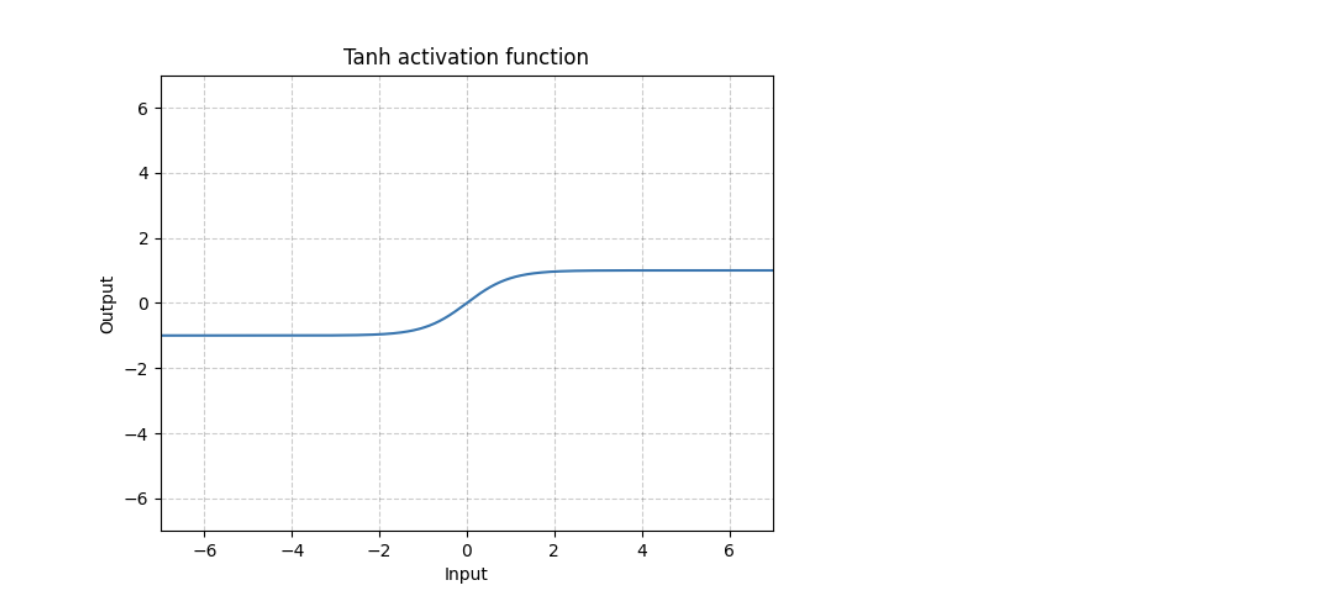**Difference between tanh and sigmoid **— Apart from the difference in the range of these activation functions, tanh function is symmetric around the origin, whereas the sigmoid function is not.

Both sigmoid and tanh pose vanishing gradient problems when used as activation functions in neural networks.

**3. ReLU(Rectified Linear Unit)- **It is the most popular activation function.• Outputs the same value for a positive value and zeroes out negative values.
• It is very fast to compute (given the simplicity of logic), thus improving training time.
• ReLU does not pose vanishing gradient problem.
• It does not have a maximum value.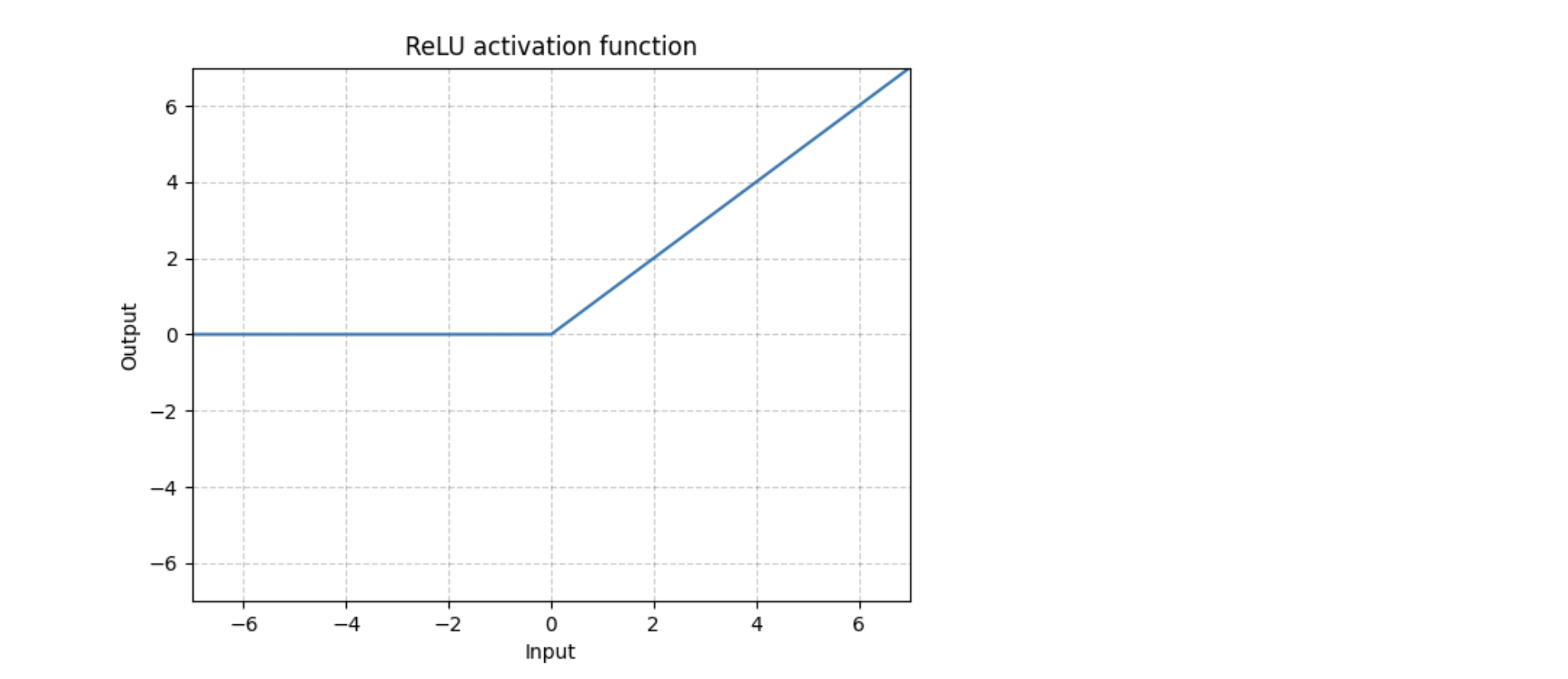There are different variations of ReLU that are available like LeakyReLU, SELU, ELU, SReLU. Still, ReLU is widely used as it is simple, fast, and efficient

#neural-networks #activation-functions #deep-learning #convolutional-network #relu1595818440

## Neural Networks from Scratch - P.5 Hidden Layer Activation Functions

Neural Networks from Scratch book, access the draft now: https://nnfs.io

NNFSiX Github: https://github.com/Sentdex/NNfSiX